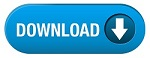Home » SSC » Model Questions for Scientific Assistant (IMD) Exam (Paper-2) PHYSICS – Set 1

# Model Questions for Scientific Assistant (IMD) Exam (Paper-2) PHYSICS – Set 1## Q- 1. An athlete performs a high jump. As she lands, she bends her knees as she reaches the ground. This increases the time of impact. The result is to ___ the force that her body experiences.

(A) Increase
(B) Keep the same
(C) Reduce
(D) The answer depends on how high she jumped

## Q- 2. A canon ball travelling at 10 m/s has a momentum of 50 kg m/s. What is the mass of the ball?

(A) 5 kg
(B) 50 kg
(C) 20 kg
(D) none of the above

## Q- 3. A car travelling at 10 km/hr runs into a haystack and comes to a stop. This is less dangerous than if the car had driven into a concrete wall. This is because driving into the haystack results in

(A) Increased time of impact and reduced impact force
(B) Increased impulse
(C) The same impulse
(D) Reduced change in momentum

## Q-4. Enables a system to do work.

(A) Work
(B) Power
(C) Energy
(D) Potential energy

## Q- 5.  Energy of a body that is moving, disregarding any energy due to position.

(A) Work
(B) Power
(C) Potential energy
(D) Kinetic energy

### Q- 6. Work done per unit time.

(A) Work
(B) Power
(C) Energy
(D) Potential energy

## Q- 7. Force multiplied by distance moved by the object upon which the force is acting.

(A) Work
(B) Power
(C) Energy
(D) Potential energy

## Q-8.  Work done on an object is equal to the change in kinetic energy of the object.

(A) Work-energy theorem
(B) Power
(C) Energy
(D) Potential energy

## Q- 9. A device that increases, decreases, or changes the direction of a force.

(A) Work
(B) Machine
(C) Energy
(D) Potential energy

## Q-10. Energy can not be created or destroyed by any physical entity.

(A) Work
(B) Power
(C) Conservation of energy
(D) Potential energy

#